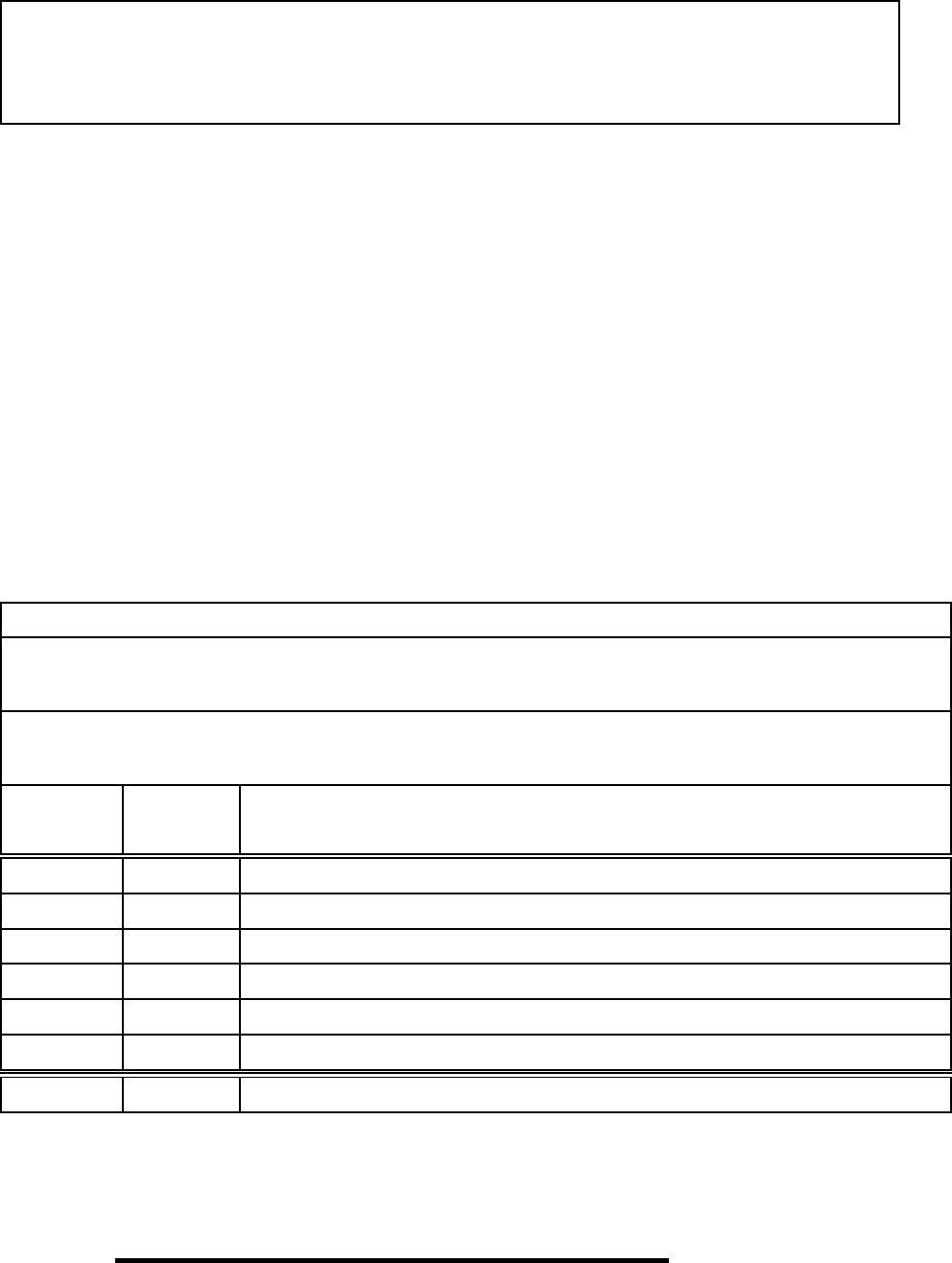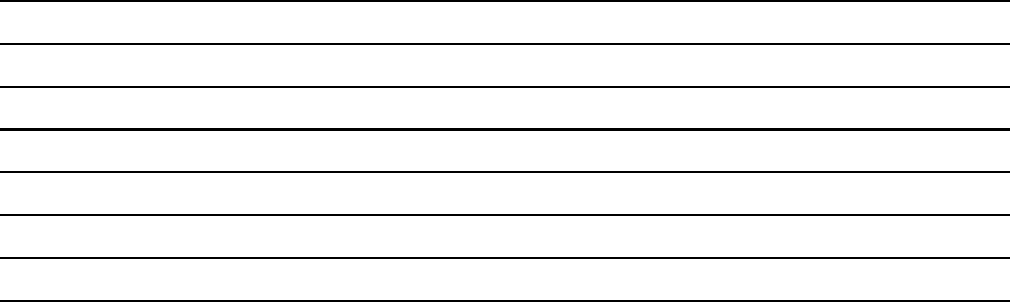Class Notes (1,100,000)
US (480,000)
WUSTL (3,000)
E81 CSE (40)
coles (4)
Lecture 16

# Computer Science And Engineering CSE 232 Lecture Notes - Lecture 16: Binary Heap, Linear Search, Linked ListExam

Department
Computer Science And Engineering
Course Code
Computer Science And Engineering CSE 232
Professor
coles
Lecture
16

This preview shows pages 1-3. to view the full 11 pages of the document.CSE 247 Data Structures and Algorithms Fall 2016
Exam I
Given: 13 October 2016 Due: End of session
This exam is closed-book, closed-notes. No electronic devices or resources of any kind are
allowed. The exception is the “cheat sheet” as described on our course web pages on which you
may have notes to consult during the exam.
Your work mu st b e legibl e. Work that is di cult to read will receive no credit. Do not dwell over
punctuation or exact syntax in code; however, be sure to indent your code to show its structure.
You must sign the pledge b elow for your exam to count. Any cheating will cause the students
involved to receive an F for this course. Other action may be taken.
Your wor k must b e completed on these pages. There are blan k p ages at the end of the exam if
you need them. Don’t spend too much time staying stuck on a question: move on and return to
the question later.
You must ﬁll in your identifying information correctly. Whenyoureachthispointinthe
instructions, please give the professor or TA a meaningful glance.
Print clearly the following information:
Name (print clearly):
Student 6-digit ID (print really clearly):
Number Points Points
120
210
310
420
520
620
Total 100
Pledge: On my honor, I have neither given nor received any unauthorized aid on this exam.
Signed:
(Be sure you ﬁlled in your information in the box above!)

Only pages 1-3 are available for preview. Some parts have been intentionally blurred.CSE 247 (Exam I) –2– Due by End of session
1. (20 points) In this problem, you may assume the log function is computed with any
base you like, but state the base you are assuming.
(a) (5 points) nlog n=O(g(n)) for which of the following deﬁnitions of g(n)?
Circle all that apply:
g(n)=1
g(n)=n
g(n)=nlog n
g(n)=n2nlog n
g(n)=n2log n
(b) (5 points) nlog n=(g(n)) for which of the following deﬁnitions of g(n)?
Circle all that apply:
g(n)=1
g(n)=n
g(n)=nlog n
g(n)=n2nlog n
g(n)=n2log n
(c) (5 points) nlog n=Θ(g(n)) for which of the following deﬁnitions of g(n)?
Circle all that apply:
g(n)=1
g(n)=n
g(n)=nlog n
g(n)=n2nlog n
g(n)=n2log n
(d) (5 points) Using limits and L’Hˆopital’s rule, provide a proof that
1000 log n=Θ(log (nc))
where cis some positive constant (independent of nand c<n).

Only pages 1-3 are available for preview. Some parts have been intentionally blurred.CSE 247 (Exam I) –3– Due by End of session
2. (10 points) For each of the program fragments below, let T(n)represent,worst-case,
the execution time of that program fragment in terms of n, an input value to each
fragment.
(a) (1 points) Fragment:
for i=1 to n
for j=1 to n
a[i][j] = b[i][j] + c[i][j]
end
end
Worst-case, the above fragment takes Θ() time.
(b) (3 points) Fragment:
for i=1 to n
int[] array = new int;
end
Worst-case, the above fragment takes Θ() time.
(c) (3 points) Fragment:
for i=1 to n
int[] array = new int[i];
end
Worst-case, the above fragment takes Θ( ) time.
(d) (3 points) Fragment:
for i=1 to n
if Math.random() < 0.0000001 // very unlikely this is true
for j=1 to n
a[i][j] = b[i][j] + c[i][j]
end
end
end
Worst-case, the above fragment takes Θ() time.